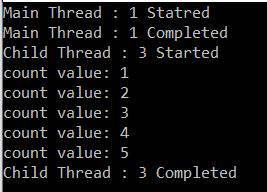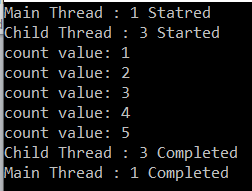# Task-based Asynchronous Programming in C#

## Task-based Asynchronous Programming in C#

Before understanding theory i.e. what is Task and what are the benefits of using Task, let us first discuss how to create and use Task in C#.

##### Working with Task in C#:

Note: In general, the Task class will always represent a single operation and that operation will be executed asynchronously on a thread pool thread rather than synchronously on the main thread of the application. If this is not clear at the moment then don’t worry we will discuss this in practice.

##### Example: Using the Task class and Start method in C#

In the below example, we are creating the task object by using the Task class and then start executing it by calling the Start method on the Task object.

```using System;

{
class Program
{
static void Main(string[] args)
{
}

static void PrintCounter()
{
for (int count = 1; count <= 5; count++)
{
Console.WriteLine(\$"count value: {count}");
}
}
}
}```

In the above example, we created the task object i.e. task1 using the Task class and then call the Start method to start the task execution. Here, task object task1 will create a new child thread to execute the defined functionality asynchronously on a thread pool thread. So, when you run the above application, you will get the following output.As you can see in the above output, two threads are used to execute the application code. The main thread and the child thread. And you can observe both threads are running asynchronously.

##### Example: Creating a task object using Factory Property

In the following example, we are creating the task object using the Factory property which will start automatically.

```using System;

{
class Program
{
static void Main(string[] args)
{
}

static void PrintCounter()
{
for (int count = 1; count <= 5; count++)
{
Console.WriteLine(\$"count value: {count}");
}
}
}
}
```

It will give you the same output as the previous example. The only difference between the previous example and this example is here we creating and running the thread using a single statement.

##### Example: Creating a Task object using the Run method

In the following example, we are creating a task by using the Run method of the Task class.

```using System;

{
class Program
{
static void Main(string[] args)
{
}

static void PrintCounter()
{
for (int count = 1; count <= 5; count++)
{
Console.WriteLine(\$"count value: {count}");
}
}
}
}
```

So, we have discussed three different ways to create and start a task in C#. From a performance point of view, the Task.Run or Task.Factory.StartNew methods are preferable to create and schedule the tasks. But, if you want to the task creation and scheduling separately, then you need to create the task separately by using Task class and then call the Start method to schedule the task execution for a later time.

##### Task using Wait in C#:

If you want to make the main thread execution wait until all child tasks are completed, then you need to use the Wait method of the Task class. The Wait method of the Task class will block the execution of other threads until the assigned task has completed its execution.

In the following example, we are calling the Wait() method on the task1 object to make the program execution wait until task1 completes its execution.

```using System;

{
class Program
{
static void Main(string[] args)
{
{
PrintCounter();
});
}

static void PrintCounter()
{
for (int count = 1; count <= 5; count++)
{
Console.WriteLine(\$"count value: {count}");
}
}
}
}
```

As you can see in the above code, we are calling the Wait() method on the task object i.e. task1. So, the main thread execution will wait until the task1 object completes its execution. Now run the application and see the output as shown in the below image.So as of now, we have discussed how to work with threads using different approaches. Now let us discuss what is Task and why should we use Task?

##### What Is A Task In C#?

A task in C# is used to implement Task-based Asynchronous Programming and was introduced with the .NET Framework 4. The Task object is typically executed asynchronously on a thread pool thread rather than synchronously on the main thread of the application.

##### What is a Thread pool in C#?

A Thread pool in C# is a collection of threads that can be used to perform a number of tasks in the background. Once a thread completes its task, then again it is sent to the thread pool, so that it can be reused. This reusability of threads avoids an application to create a number of threads which ultimately uses less memory consumption.

##### Why do we need to use a Task In C#?

Tasks in C# basically used to make your application more responsive. If the thread that manages the user interface offloads the works to other threads from the thread pool, then it can keep processing user events which will ensure that the application can still be used.

That’s it for today. In the next article, I am going to discuss how to return a value from a task in C# with some examples. Here, in this article, I try to explain Task-based Asynchronous Programming in C# using the Task class. I hope you understood how to create and use Task class objects in C#.

### 3 thoughts on “Task-based Asynchronous Programming in C#”

1.In the 1st example you provided, the main thread is getting executed 1st, completed then the child thread is executing. This is Synchronous in nature. So, in that case what is the use of the task? Can you please provide a little more detail?

1.No, It’s not like that, Task will be executed asynchronously in the background. The main thread is also executed parallelly and may or may not be completed before child threads, it is simply unpredictable. If you try to do some time taking task in the main thread, you will get to know that child threads are completed before Main thread.